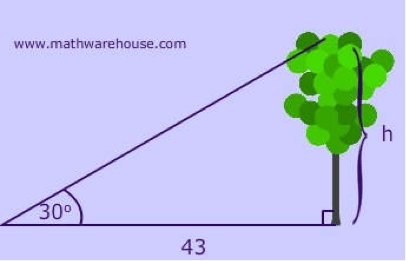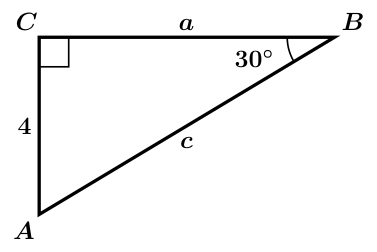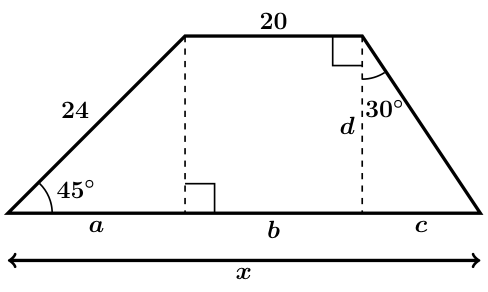# GEOM 2 | Lesson 2 | Practice (Special Right Triangles)# Special Right Triangles

Apply the theorems now to solve the following problems.  Note that figures are not drawn to scale.

1. What is the measure of the other 2 sides in this triangle?1. Find the height of the tree.1. Find the missing sides in the triangle.1. Suzie sees a plane at 10,000 feet above sea level headed straight for her boat at a 30 degree angle of depression. What is the horizontal distance between her and the plane? And how much further does the plane have to travel before it hits her boat?1. Find all of the unknowns in this figure using special right triangles.Check solutions here.